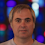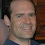## Monday, September 17, 2012

### Imagining Imaginary Probabilities

In the year 4000BC my great-great-...-great grandmother tried to solve (in today's terms) the equation
x2 + 2x + 2 = 0
She discovered that if it had a solution then there would be a number a such that a2=-1. Since there clearly was no such number, the equation had not solution. She missed her chance to (depending on your viewpoint) discover or invent complex numbers.

Fast Forward 6012 years.

In the year 2012 I wondered: is there a probability p such that if you flip a coin that has prob(H)=p twice the prob that you get HT is 1/2? This leads to
p(1-p)=1/2
If you solve this you get p=(1+i)/2. Hence there is no such coin. WAIT A MINUTE! I don't want to miss the chance that my great...great grandmother missed! In the real world you can't have a coin with prob(H) = (1+i)/2. But is there some meaning to this?

More generally, for any 0 ≤ d ≤ 1 there is a p ∈ C (the complex numbers) such that prob(HT)=d.'' The oddest case (IMHO) was to take d=1. You then get that if a coin has prob(H)=(1+\sqrt(-3))/2 then prob(HT)=1. Does that mean it always happens? No since prob(TH)=1. Do the probs of HH, HT, TH, TT add up to 1? Yes they do since some are negative.

Is there an interpretation or use for this? I know that quantum mechanics uses stuff like this. Could examples like this be good for education? Are there non-quantum examples of the uses of this thatcould be taught in a discrete math course?

1.If I recall correctly, imaginary probabilities figured in Isaac Asimov's Foundation Trilogy.

2.You were on your way to re-discovering quantum information until you decided to insist on the solution being "non-quantum". :)

3.FYI: http://www.scottaaronson.com/democritus/lec9.html

4.Last year I heard a nice talk by Persi Diaconis on a probability theory with negative numbers and the analogues of standard theorems (such as the central limit theorem) one can prove in this theory.

An online version seems to be here:
http://vimeo.com/29445127

5.Why just have probability? Complex measure theory lets you have imaginary masses, distances, volumes, etc. Everything you need for a surreal existence.

6.With some effort you could probably extend this to complex surreal numbers.
http://en.m.wikipedia.org/wiki/Surreal_number

7.If the "interpretation" is that probabilities p are not constant, but represent expectations over some function y of unwritten parameters t (e.g. a time series), then at least it's consistent to have both E[y_t = H] = p_t = 1/2, and E[y_t = H and y_{t+1} = T] = p_t (1 - p_{t+1}) = 1/2.

But at the moment I can't think of an interpretation for an imaginary solution to the arbitrary equation of two elements of the series that isn't nonsense, without introducing more arbitrary structure over the parameters. (Like in QM.)

8.This reminds me of the idea of a "half coin." A half coin is one that if you flip it twice you get exactly what a single coin toss looks like. This turns out to have a fairly precise definition--a sensiable mean, variance, moment generating function, central limit theorem, etc. What it doesn't have is probabilities that live between zero and one!